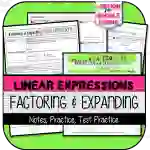Math > Algebra | Grade 7 | Activities

# Factoring & Expanding Linear Expressions NOTES & PRACTICEAttributes
Subject

Algebra

Types

Activities

File

PDF

Editable
No
RatingThis resource was developed to PARTIALLY meet the requirements of the 7th Grade Expressions & Equations Standards below:

CCSS.MATH.CONTENT.7.EE.A.1
Apply properties of operations as strategies to add, subtract, factor, and expand linear expressions with rational coefficients.

## What's Included

This resource contains the following items:

1) Factoring & Expanding (Distributive Property*) Linear Expressions NOTES & PRACTICE

2) Adding & Subtracting Linear Expressions TEST PRACTICE (Printable and version for Google Forms)

3) Answer Key to All Parts

*This lesson assumes students have already been introduced to the distributive property using rational numbers & integer rules.

## Resource Tags

factoring distributive property notes linear expressions

## 0 Reviews

### you may also like...

Check out these other great products